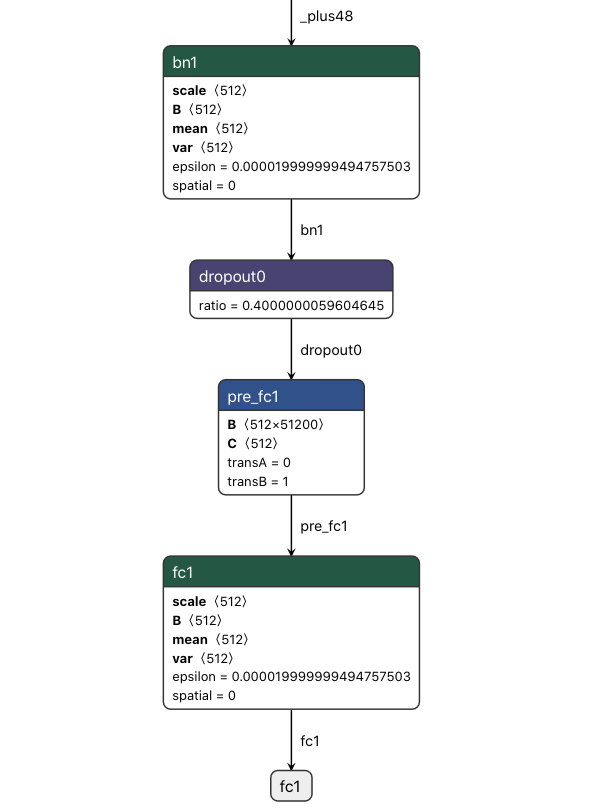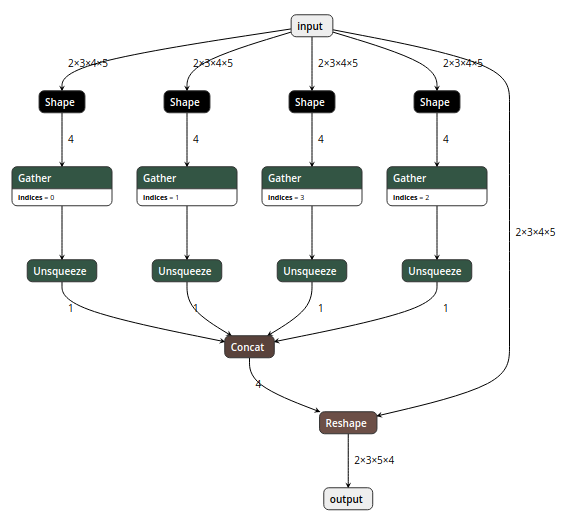bindog study Machine Learning & Security

# 深度学习模型转换与部署那些事(含ONNX格式详细分析)

2020-03-13

## 第一部分：ONNX结构分析与修改工具

### ONNX结构分析

ONNX结构的定义基本都在这一个onnx.proto文件里面了，如何你对protobuf不太熟悉的话，可以先简单了解一下再回来看这个文件。当然我们也不必把这个文件每一行都看明白，只需要了解其大概组成即可，有一些部分几乎不会使用到可以忽略。

• ModelProto
• GraphProto
• NodeProto
• AttributeProto
• ValueInfoProto
• TensorProto

### ONNX的兼容性问题• https://github.com/onnx/models/issues/156
• https://github.com/microsoft/onnxruntime/issues/2175## 第二部分：各大深度学习框架如何转换到ONNX？

（需要说明的是，由于深度学习领域发展迅速，本文提到的几个框架也在快速的迭代过程中，所以希望本文提到的一些坑和bug在未来的版本当中能够逐一解决，也希望大家永远不要踩本文所提到的那些坑）

### MXNet转换ONNX

MXNet官方文档给出了一个非常简单的例子展示如何转换

import mxnet as mx
import numpy as np
from mxnet.contrib import onnx as onnx_mxnet
import logging
logging.basicConfig(level=logging.INFO)

# Download pre-trained resnet model - json and params by running following code.
path='http://data.mxnet.io/models/imagenet/'

sym = './resnet-18-symbol.json'
params = './resnet-18-0000.params'

# Standard Imagenet input - 3 channels, 224*224
input_shape = (1,3,224,224)

# Path of the output file
onnx_file = './mxnet_exported_resnet50.onnx'

# Invoke export model API. It returns path of the converted onnx model
converted_model_path = onnx_mxnet.export_model(sym, params, [input_shape], np.float32, onnx_file)


count_include_pad = 1 if attrs.get("count_include_pad", "True") in ["True", "1"] else 0
# ...
# ...
# ...
elif pool_type == "avg":
node = onnx.helper.make_node(
pool_types[pool_type],
input_nodes,  # input
[name],
kernel_shape=kernel,
strides=stride,
name=name
)


### TensorFlow模型转ONNX

tf的模型转换ONNX已经有现成的转换工具，https://github.com/onnx/tensorflow-onnx，先将tf的模型freeze_graph之后得到pb文件，再利用该转换工具即可转换为onnx模型

freeze_graph的方式网上有很多版本，我这里用的是一个老版本的方法(tensorflow==1.8.0)


import network

input_size = (224, 224)
ckpt_model_path = "./model.ckpt"
pb_model_path = "./model.pb"
output_node_name = "your model output name"

graph = tf.Graph()
with graph.as_default():
placeholder = tf.placeholder(
dtype=tf.float32, shape=[None, input_size, input_size, 3], name="pb_input"
)
output = network(placeholder)

# your can get all the tensor names if you do not know your input and output name in your ckpt with this code
# nl = [n.name for n in tf.get_default_graph().as_graph_def().node]
# for n in nl:
#     print(n)

saver = tf.train.Saver()
sess = tf.Session(
config=tf.ConfigProto(
gpu_options=tf.GPUOptions(
allow_growth=True, per_process_gpu_memory_fraction=1.0),
allow_soft_placement=True
)
)
saver.restore(sess, ckpt_model_path)

output_graph_def = graph_util.convert_variables_to_constants(
sess, sess.graph_def, [output_node_name]
)
with tf.gfile.FastGFile(pb_model_path, mode="wb") as f:
f.write(output_graph_def.SerializeToString())

# you can get the input and output name of your model.pb file
# maybe a "import/" is needed to append before the name if you
# get some error
# gf = tf.GraphDef()
# nl2 = [n.name + '=>' +  n.op for n in gf.node if n.op in ('Softmax', 'Placeholder')]
# for n in nl2:
#     print(n)


python3 -m tf2onnx.convert --input xxxx.pb --inputs pb_input:0 --inputs-as-nchw pb_input:0 --outputs resnet_v2_101/predictions/Softmax:0 --output xxxx.onnx


### PyTorch模型转ONNX

import torch
import torchvision

dummy_input = torch.randn(10, 3, 224, 224, device='cuda')
model = torchvision.models.alexnet(pretrained=True).cuda()

# Providing input and output names sets the display names for values
# within the model's graph. Setting these does not change the semantics
# of the graph; it is only for readability.
#
# The inputs to the network consist of the flat list of inputs (i.e.
# the values you would pass to the forward() method) followed by the
# flat list of parameters. You can partially specify names, i.e. provide
# a list here shorter than the number of inputs to the model, and we will
# only set that subset of names, starting from the beginning.
input_names = [ "actual_input_1" ] + [ "learned_%d" % i for i in range(16) ]
output_names = [ "output1" ]

torch.onnx.export(model, dummy_input, "alexnet.onnx", verbose=True, input_names=input_names, output_names=output_names)


## 第三部分：ONNX到目标平台

ONNX实际只是一套标准，里面只不过存储了网络的拓扑结构和权重（其实每个深度学习框架最后固化的模型都是类似的），脱离开框架是没有办法直接进行inference的。大部分框架（除了tensorflow）基本都做了ONNX模型inference的支持，这里就不进行展开了。